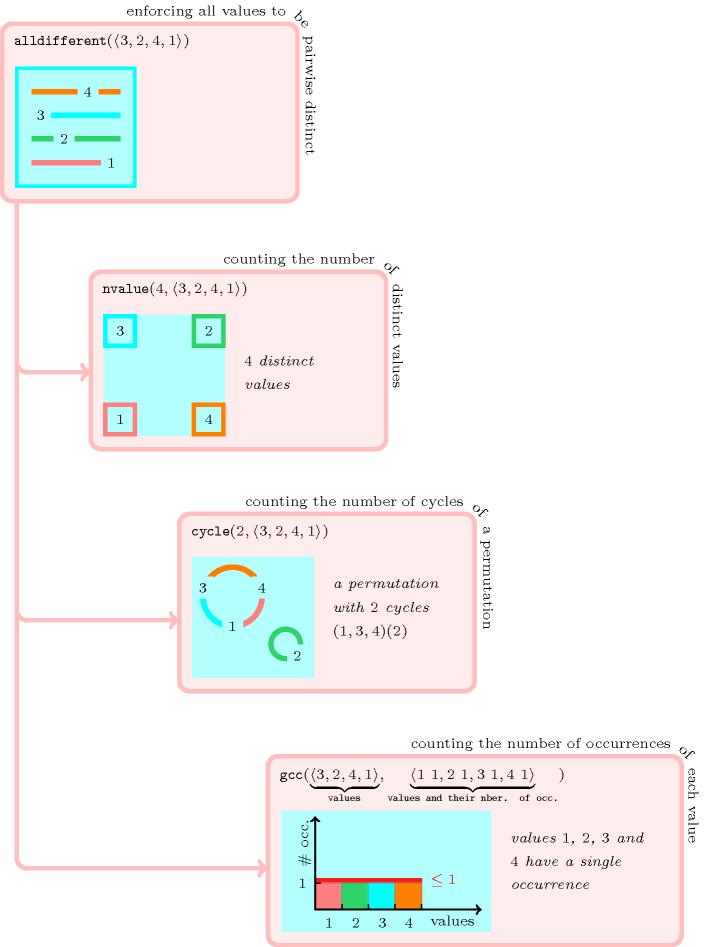### 3.7.66. Core

Denotes that a global constraint is an important constraint. In fact many constraints can been seen as variations or extensions around one of the following notions:

##### Figure 3.7.19. Three counting based generalisations of the $\mathrm{𝚊𝚕𝚕𝚍𝚒𝚏𝚏𝚎𝚛𝚎𝚗𝚝}$ constraint: the $\mathrm{𝚗𝚟𝚊𝚕𝚞𝚎}$, the $\mathrm{𝚌𝚢𝚌𝚕𝚎}$ and the $\mathrm{𝚐𝚕𝚘𝚋𝚊𝚕}_\mathrm{𝚌𝚊𝚛𝚍𝚒𝚗𝚊𝚕𝚒𝚝𝚢}$ (i.e., $\mathrm{𝚐𝚌𝚌}$) constraints; the same example $\mathrm{𝚊𝚕𝚕𝚍𝚒𝚏𝚏𝚎𝚛𝚎𝚗𝚝}$$\left(〈3,2,4,1〉\right)$ is reinterpreted with respect to the three generalisationsBoth, the $\mathrm{𝚎𝚕𝚎𝚖𝚎𝚗𝚝}$ and the $\mathrm{𝚊𝚕𝚕𝚍𝚒𝚏𝚏𝚎𝚛𝚎𝚗𝚝}$ constraints, are the most commonly used global constraints. Many core global constraints can be seen as an extension of the $\mathrm{𝚊𝚕𝚕𝚍𝚒𝚏𝚏𝚎𝚛𝚎𝚗𝚝}$$\left(〈{x}_{1},{x}_{2},\cdots ,{x}_{n}〉\right)$ constraint along one of the two following lines: